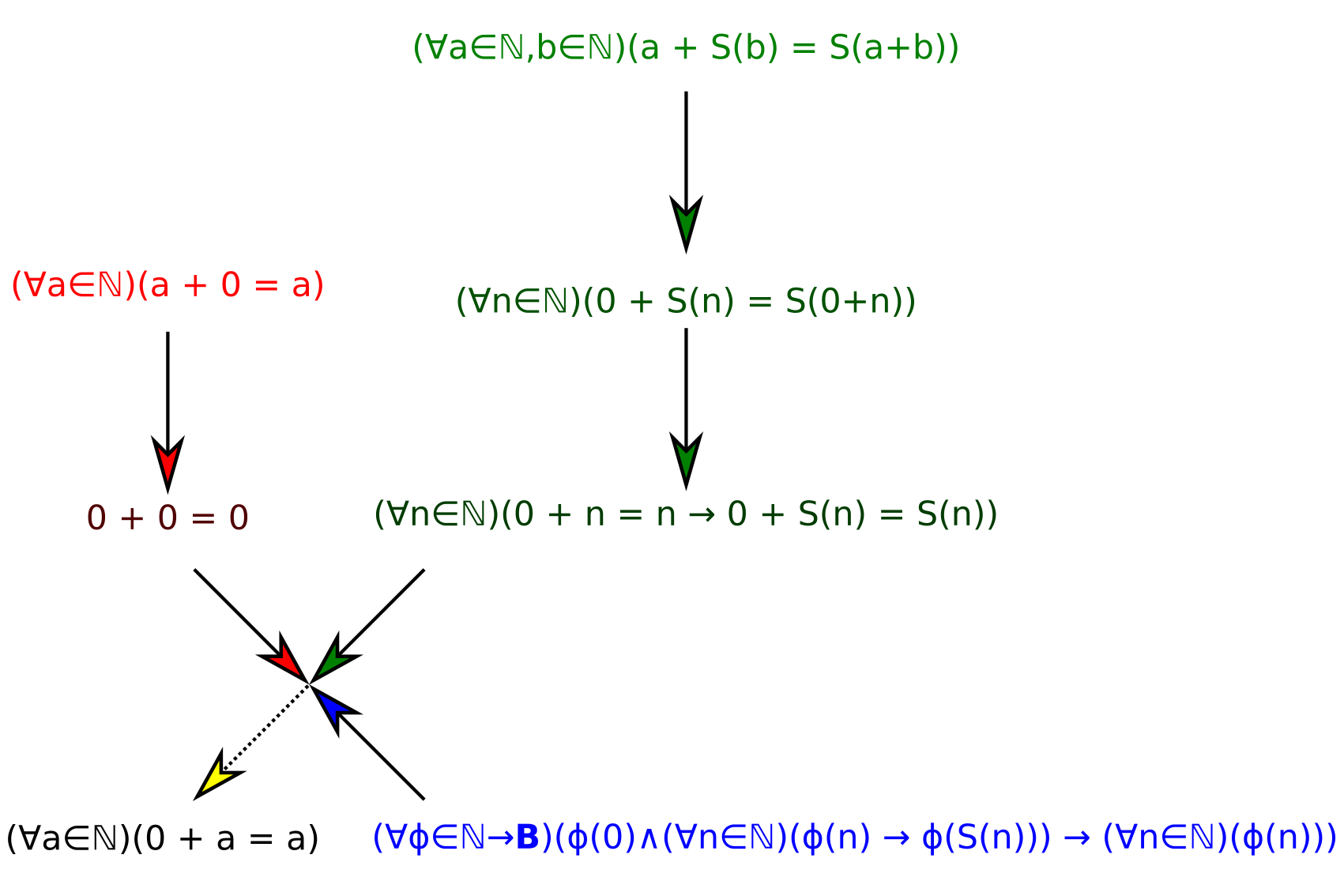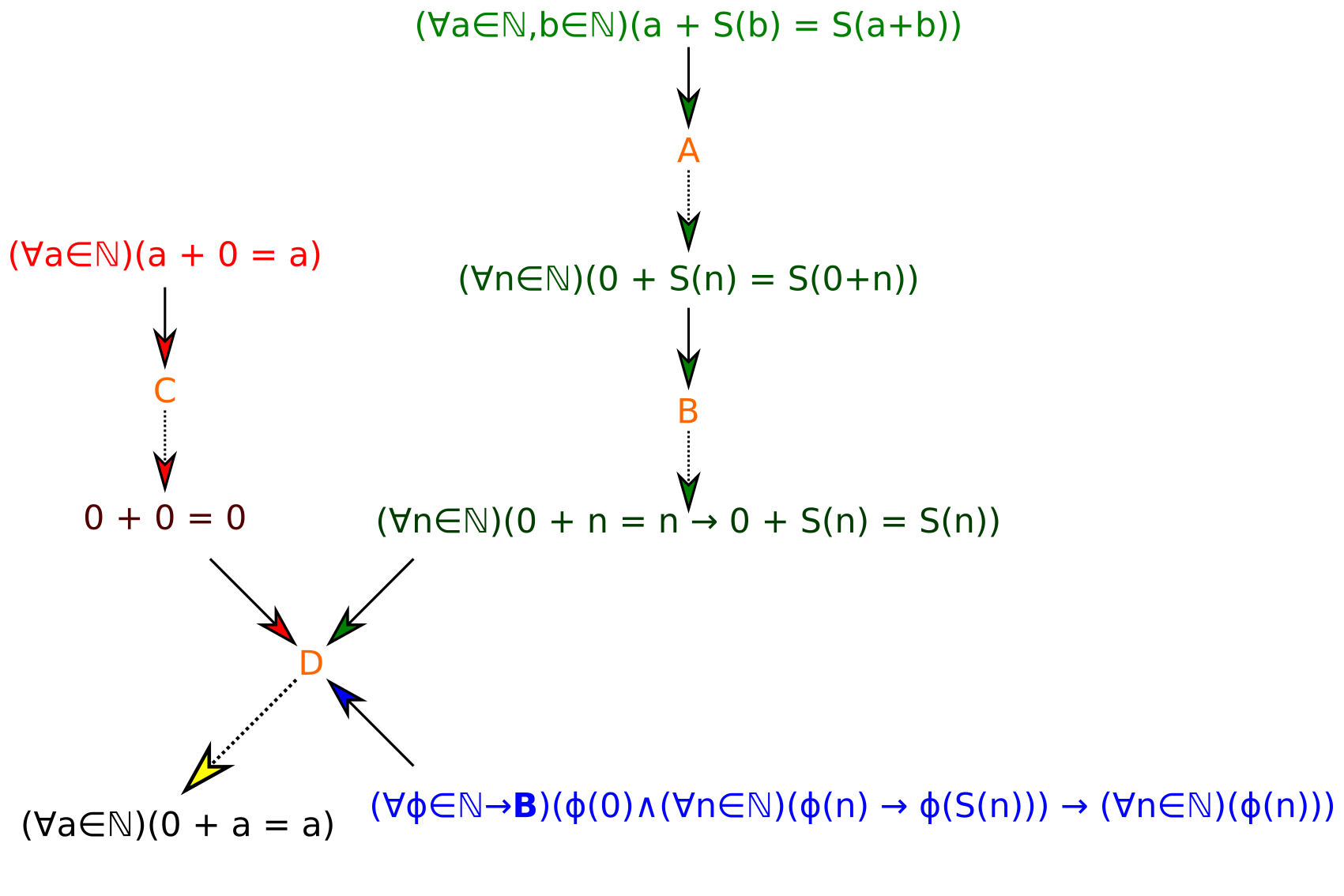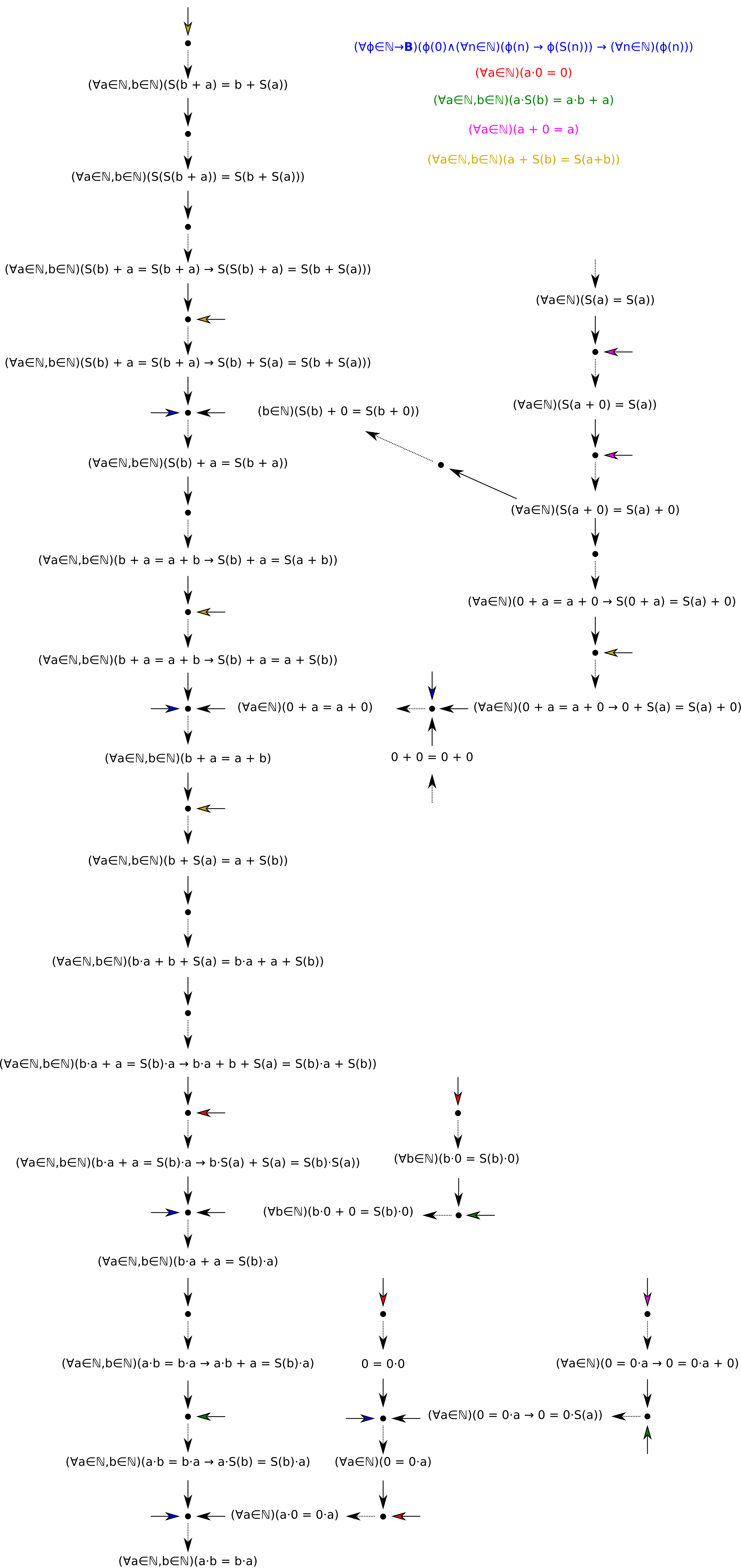# The Triviality Norm

Feynman famously said that to a mathematician, anything that was proven was trivial. This statement, while clearly a lighthearted dig at mathematics, it does have a striking ring of truth to it.

## Logical Implication

In logic, there is the concept of implication. The idea is that if $$B$$ is true whenever $$A$$ is true we can say that $$A \implies B$$. This makes sense for statements that depend on a variable. For example, we can say that $$x > 2 \implies x > 0$$.

However, on the other hand, implication, because it is just a mechanical definition based on the truth values of the inputs and not their relationship to each other, can sometimes lead to ridiculous results. For example, this implication is completely valid:

$1 = 1 \implies a + b = b + a$

Which doesn’t seem to make sense: the first is a tautology about equality and the second is a tautology about addition. Additionally, the following statement is true:

$2 = 3 \implies \text{Fermat’s Last Theorem is False}$

In both cases (truth implies truth and falsehood implies falsehood) make some degree of sense. However, the final case, falsehood implies truth, is where things start becoming weird:

$2 = 3 \implies 2 = 2$

In any case, the fact that implication in logic, unlike implication in language, does not imply (in either sense) a connection between two concepts. In fact, there is no distinction between a simple proof and a more complex one. In other words, using logical implication as a metric, anything that is proven is trivial.

## Intuitive Implication

$$\newcommand\rew{\gg}$$

We can define intuitive implication, or a rewrite, as a single logical reduction from a step to another, denoted $$A \rew B$$. For example, we have

$\neg (A \wedge B \wedge C) \rew \neg (A \wedge (B \wedge C)) \rew \neg A \vee \neg (B \wedge C) \rew \neg A \vee (\neg B \vee \neg C) \rew \neg A \vee \neg B \vee \neg C$

However, this general scheme doesn’t seem to work for more complex theorems. For example (using new lines rather than $$\rew$$),

$a + 0 = a \wedge a + S(b) = S(a + b) \wedge \text{Induction}$ $a + 0 = a \wedge a + S(b) = S(a + b) \wedge \text{Induction} \wedge 0 + 0 = 0$ $a + S(b) = S(a + b) \wedge \text{Induction} \wedge 0 + 0 = 0 \wedge (0 + n = n \implies S(0 + n) = S(n))$ $\text{Induction} \wedge 0 + 0 = 0 \wedge (0 + n = n \implies 0 + S(n) = S(n))$ $0 + n = n$

In general, this strategy doesn’t work well. In reality, a different given statement is used at every step in the process. We can represent this process as:This structure is not exactly a graph, but does have certain properties of one. We can represent each step as an implication between a tuple of statements and a result:

$(\forall a \in \mathbb N)(a + 0 = a) \rew 0 + 0 = 0$ $(\forall a \in \mathbb N, b \in \mathbb N)(a + S(b) = S(a + b)) \rew (\forall n\in\mathbb N)(0 + S(n) = S(0+n))$ $(\forall n\in\mathbb N)(0 + S(n) = S(0+n)) \rew (\forall n\in\mathbb N)(0 + n = n \implies 0 + S(n) = S(n))$ $\big(0 + 0 = 0;(\forall n\in\mathbb N)(0 + n = n \implies 0 + S(n) = S(n));(\forall\phi\in\mathbb N \to\mathbb B)(\phi(0)\wedge(\forall n\in\mathbb N)(\phi(n) \implies \phi(S(n))) \implies (\forall n \in\mathbb N)(\phi(n)))\big) \rew (\forall n \in \mathbb N)(0 + n = n)$

## The graph structure

The structure of a proof under this scheme is not quite an exact graph. A graph can take the form (using Haskell notation)

data Edge a = Edge {
from :: a,
to :: a
}
data Graph a = Graph [Edge a]


A more convenient representation (for searches, etc.) is:

data GraphNode a = GraphNode {
value :: a,
neighbors :: [GraphNode a]
}

type Graph a = [GraphNode a]


This graph, on the other hand, has asymetrical edges, with multiple start nodes for each end node. We have:

data Edge a = Edge {
from :: [a],
to :: a
}

data Graph a = Graph [Edge a]


A more convenient representation is difficult in this case, given that the transformation used above:

data Neighbor a = Neighbor {
otherDependencies :: [GraphNode a],
target :: GraphNode a
}

data GraphNode a = GraphNode {
value :: a,
neighbors :: [Neighbor a]
}

type Graph a = [GraphNode a]


As you can see, each of the neighbors needs to mantain a list of dependencies, which makes it difficult to see the whole structure of the graph. Instead, we can use edge nodes, making a graph look like this:This can be represented like this:

data GraphNode a = GraphNode {
value :: a,
contributesTo :: [EdgeNode a]
}

data EdgeNode a = EdgeNode {
from :: [GraphNode a],
to :: GraphNode a
}

type Graph a = [GraphNode a]


This ends up being a much simpler description, being a traditional graph with two types of nodes and certain restrictions.

## The Generalized Levenshtein Distance

The Levenshtein distance, or L-distance, is the minimum number of edits needed to go between two strings, where edits are defined as

1. Deleting a character
2. Inserting a character
3. Replacing a character with another character

One basic generalization is to allow for an arbitrary set of rewrite rules. This is the graph-distance of the rewrite graph, or the graph formed by using statements as nodes and logical conclusions as edges. In this case, a theorem is just two connected nodes, along with the shortest path between them.

However, this fails to take into account the two-node nature of actual proof graphs. In this case, it does not make sense to speak of a path, instead we have a tree with certain leaves, which we know as axioms. The Generalized Levenshtein distance, or GLD, is defined as the number of edges in the tree that lead from a graph node to an edge node. For example, GLD(the above proof that 0 + a = 0 given the Peano Axioms) = 6.

And there you have it, an actual way to measure the triviality of a proof.

## Appendix: A more complex proof

This section isn’t exactly necessary, but drawing complex arrow diagrams is actually pretty fun, so I decided to draw the proof that $$ab = ba$$. Note how this proof demonstrates the graph-nature of the proof. (For simplicity, I use colored arrows to refer to axioms, with a color legend at the top.)